### Circle with Arc, Chord, Inscribed Angle, Circumscribed Polygon

Illustrations of a circle with arc, chord, inscribed angle, and circumscribed about a polygon.### Arc and Chord in Circle

Illustration of circle with arc and chord.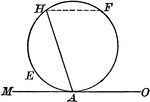### Circle With Chord and Tangent

Illustration of a circle showing an angle included by a tangent and a chord drawn from the point of…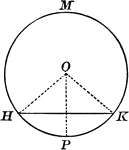### Circle with Chord and Triangle

Illustrations of a circle with a chord and triangle inside.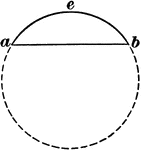### Chord of Circle

Illustration of a circle with the chord eb drawn and labeled.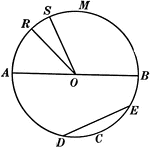### Chords, Diameters, and Radii of a Circle

Illustration of a circle with diameter AB. Radii are RO, SO, AO, and BO. ED is a chord.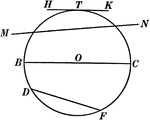### Chords, Secants, Diameters, and Tangents of a Circle

Illustration of a circle with diameter BC, chord DF, secant MN, and tangent HK.### Circle With Diameter and Chord

Illustration of a circle with diameter and chord drawn. A diameter perpendicular to a chord bisects…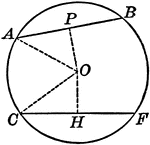### Circle With Equal Chords

Illustration of a circle with equal chords, which are equally distant from the center.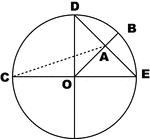### Parts of Circle

Illustration of circle with arc, chord, diameter and radius.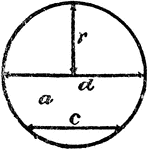### Radius, Diameter, and Chord In A Circle

An illustration showing a circle with radius r, diameter d, and chord c.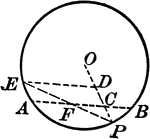### Circle and Triangle

Circle with triangle and chords.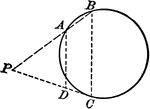### Circle and Triangle

Circle with triangle and chords.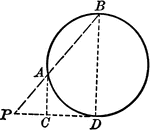### Circle and Triangle

Circle with triangle and chords.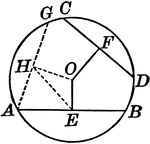### Circle With Unequal Chords

Illustration of a circle with unequal chords, which are unequally distant from the center.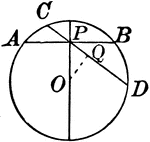### Circle With Various Chords

Illustration showing a circle with various chords drawn.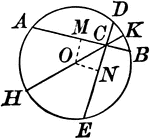### Circle With Various Chords

Illustration showing a circle with various chords drawn.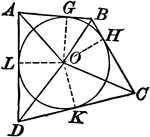### Circle With Various Chords And Tangents

Illustration showing a circle with various chords and tangents drawn.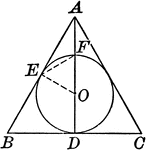### Circle With Various Chords And Tangents

Illustration showing a circle with various chords and tangents drawn.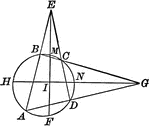### Circle With Inscribed Quadrilateral and Triangles Formed

Illustration showing a circle with an inscribed quadrilateral and triangles formed by extended chords.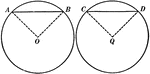### Equal Chords in Equal Circles Theorem

Illustration used to show that "In equal circles, or in the same circle, if two chords are equal, they…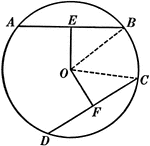### Equal Chords in Equal Circles Theorem

Illustration used to show that "In equal circles, or in the same circle, if two chords are equal, they…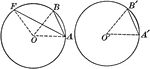### Equal Circles With Radii and Chords

Illustrations of 2 equal circles with radii drawn.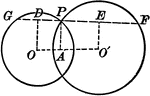### Intersecting Circles

Intersecting circles with chords and radii.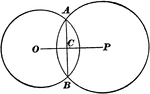### Intersecting Circles

Illustration of intersecting circles. "If two circles intersect each other, the line joining their centers…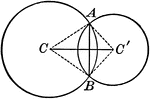### Intersecting Circles With a Common Chord

Illustration of intersecting circles with a common chord.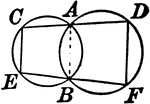### Two Intersecting Circles With Lines

Illustration showing 2 intersecting circles with a line drawn through each point of intersection terminated…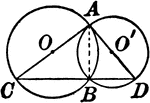### Two Intersecting Circles With Lines

Illustration showing 2 intersecting circles with a lines drawn that form a triangle.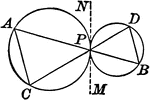### Tangent Circles With Chords

Illustration showing 2 circles with that touch each other and two lines drawn through the point of contact…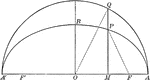### Corresponding Points in an Ellipse and Circle

Illustration of half of an ellipse. "The ordinates of two corresponding points in an ellipse and its…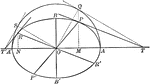### Conjugate Diameters of an Ellipse

Illustration showing that if one diameter is conjugate to a second, the second is conjugate to the first.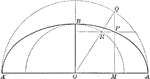### Construction of an Ellipse

Illustration of half of an ellipse and its auxiliary circle used to construct an ellipse by points,…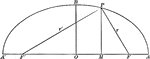### Focal Radii of an Ellipse

Illustration of half of an ellipse. "If d denotes the abscissa of a point of an ellipse, r and r' its…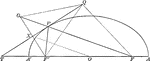### Line Bisecting Angle Between Focal Radii on Ellipse

Illustration of half of an ellipse. "If through a point P of an ellipse a line is drawn bisecting the…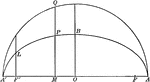### Ordinate and Major Axis of Ellipse

Illustration of half of an ellipse. The square of the ordinate of a point in an ellipse is to the product…### Parallel Tangents to an Ellipse

Illustration showing that tangents drawn at the ends of any diameter are parallel to each other.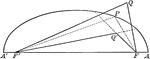### Point Distances to Foci on Ellipse

Illustration of half of an ellipse. "The sum of the distances of any point from the foci of an ellipse…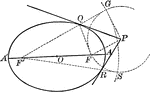### Tangent From External Point to an Ellipse

Illustration of how to draw a tangent to an ellipse from an external point.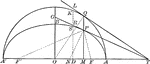### Tangents to an Ellipse

Illustration showing the tangents drawn at two corresponding points of an ellipse and its auxiliary…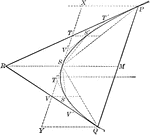### Tangents and Chords of a Parabola

Illustration showing that the area of a parabolic segment made by a chord is two thirds the area of…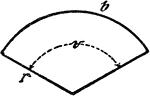### Circle Sector

An illustration showing a circle sector with radius r, center/central angle v, and length of circle…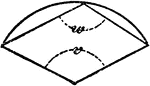### Circle Sector

An illustration showing a circle sector with center/central angle v and polygon angle w.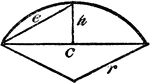### Circle Sector

An illustration showing a circle sector with height of segment h and radius r.### Semicircle With Chords and Radii

Illustration of a semicircle with chords and radii.